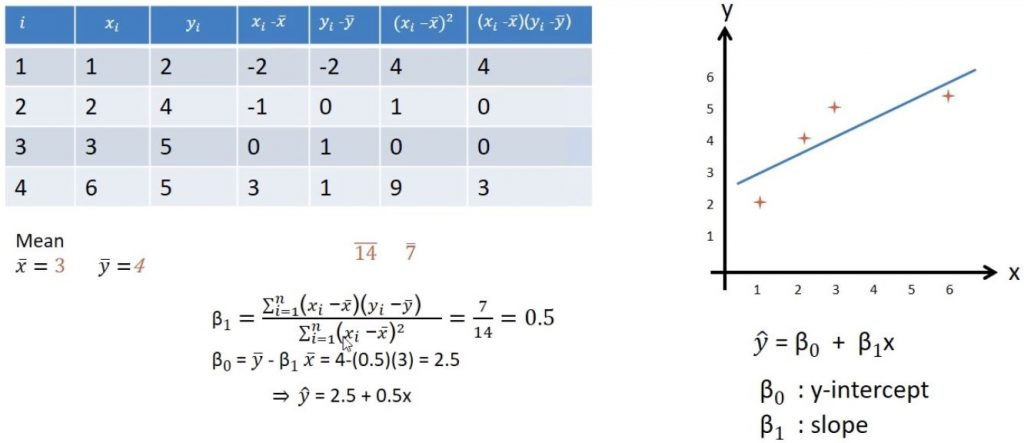# Linear regression example

I was looking for a simple example of a regression and how to calculate it by hand. I found this one: least squares example.

the main formula to calculate the linear regression is   y = Ḇo + Ḇ1xcontinue learning the basis !